[Deep Learning] 正则化

LP范数

LP范数不是一个范数，而是一组范数，其定义如下：

$$||x||_{p} = (\sum_{i}^{n}x_{i}^p)^{\frac{1}{p}}$$

$p$的范围是$[1, \infty)$。$p$在$(0,1)$范围内定义的并不是范数，因为违反了三角不等式。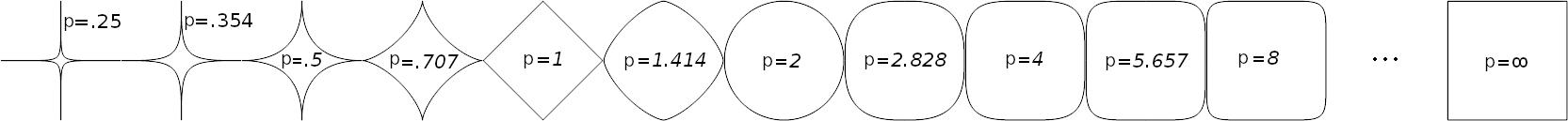L0范数表示向量中非零元素的个数，用公式表示如下：

$$||x||_{0} = \# (i | x_{i}\ne 0)$$

L1范数

$$||x||_{1} = \sum_{i}^{n} |x_{i}|$$

• 特征选择
• 可解释性

L2范数

L2范数是最熟悉的，它就是欧几里得距离，公式如下：

$$||x||_{2} = \sqrt{\sum_{i}^{n}(x_{i})^2}$$

L2范数有很多名称，有人把它的回归叫“岭回归”（Ridge Regression），也有人叫它“权值衰减”（Weight Decay）。以L2范数作为正则项可以得到稠密解，即每个特征对应的参数$w$都很小，接近于0但是不为0；此外，L2范数作为正则化项，可以防止模型为了迎合训练集而过于复杂造成过拟合的情况，从而提高模型的泛化能力。

L1范数和L2范数的区别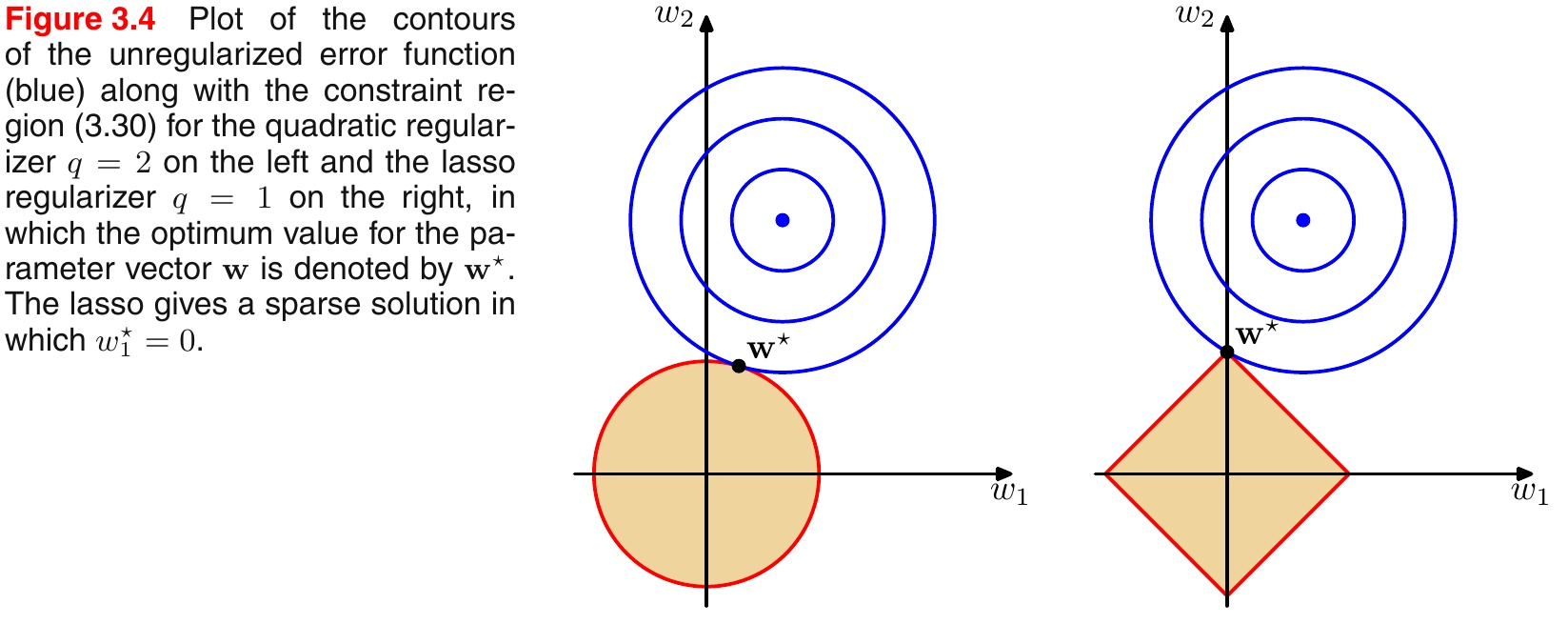• L1范数相当于加入了一个Laplacean先验；
• L2范数相当于加入了一个Gaussian先验。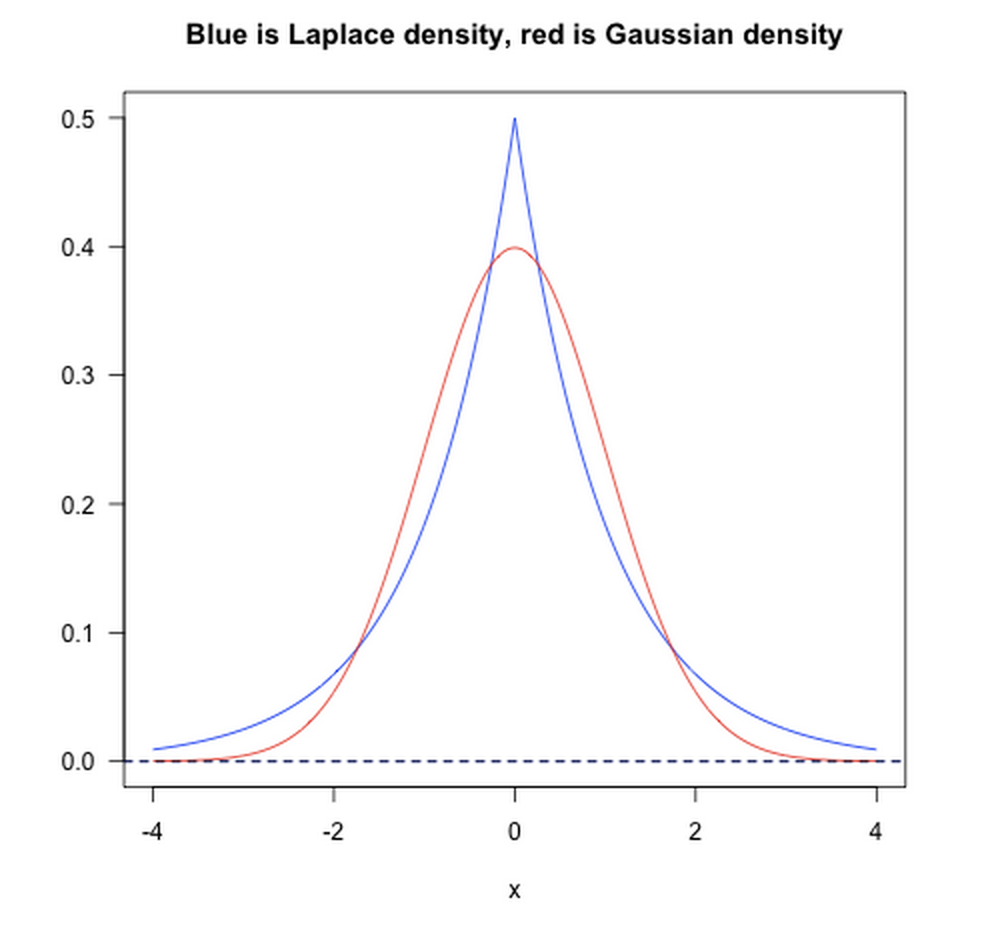Dropout

Dropout是深度学习中经常采用的一种正则化方法。它的做法可以简单的理解为在DNNs训练的过程中以概率$p$丢弃部分神经元，即使得被丢弃的神经元输出为0。Dropout可以实例化的表示为下图：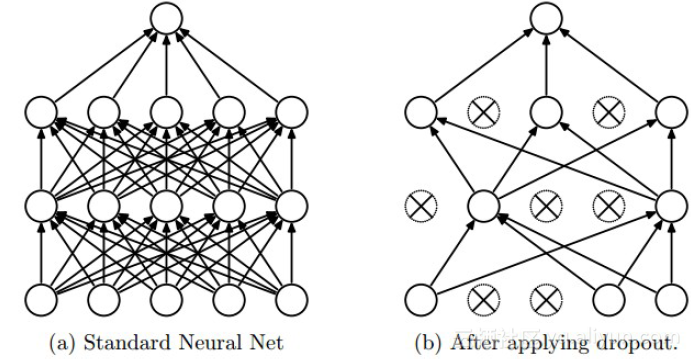• 在Dropout每一轮训练过程中随机丢失神经元的操作相当于多个DNNs进行取平均，因此用于预测时具有vote的效果。
• 减少神经元之间复杂的共适应性。当隐藏层神经元被随机删除之后，使得全连接网络具有了一定的稀疏化，从而有效地减轻了不同特征的协同效应。也就是说，有些特征可能会依赖于固定关系的隐含节点的共同作用，而通过Dropout的话，就有效地组织了某些特征在其他特征存在下才有效果的情况，增加了神经网络的鲁棒性。

Batch Normalization

BN的基本思想其实相当直观，因为神经网络在做非线性变换前的激活输入值（$X=WU+B$，$U$是输入）随着网络深度加深，其分布逐渐发生偏移或者变动（即上述的covariate shift）。之所以训练收敛慢，一般是整体分布逐渐往非线性函数的取值区间的上下限两端靠近（对于Sigmoid函数来说，意味着激活输入值$X=WU+B$是大的负值或正值），所以这导致后向传播时低层神经网络的梯度消失，这是训练深层神经网络收敛越来越慢的本质原因。而BN就是通过一定的规范化手段，把每层神经网络任意神经元这个输入值的分布强行拉回到均值为0方差为1的标准正态分布，避免因为激活函数导致的梯度弥散问题。所以与其说BN的作用是缓解covariate shift，倒不如说BN可缓解梯度弥散问题。

归一化、标准化 & 正则化

• 把数变为(0, 1)之间的小数
• 把有量纲的数转化为无量纲的数

$$x^{'} = \frac{x-\min(x)}{\max(x)-\min(x)}$$

$$x^{'}=\frac{x-\mu}{\sigma}$$

• 提升模型精度：归一化后，不同维度之间的特征在数值上有一定比较性，可以大大提高分类器的准确性。
• 加速模型收敛：标准化后，最优解的寻优过程明显会变得平缓，更容易正确的收敛到最优解。如下图所示：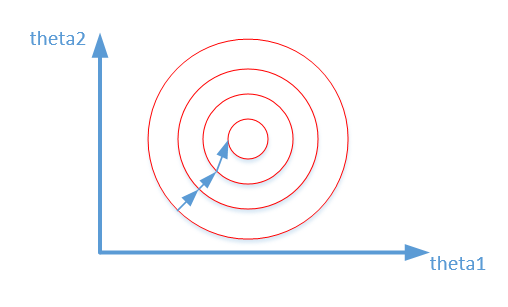Reference

1. Andrew Ng深度学习教程

<如果你觉得本文还不错，对你的学习带来了些许帮助，请帮忙点击右下角的推荐>

posted @ 2018-06-30 17:01  Poll的笔记  阅读(8761)  评论(0编辑  收藏  举报